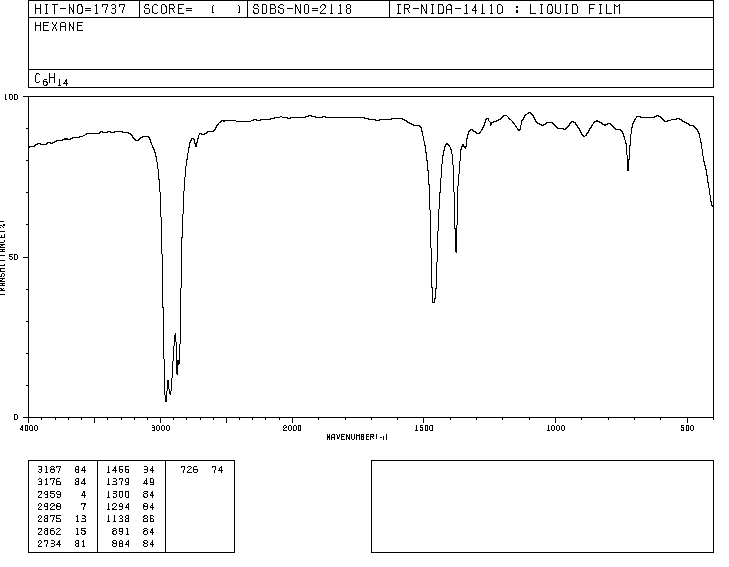Infrared radiation photons are absorbed by the bonds of a molecule, passing to higher vibrational levels.Each type of bond absorbs infrared radiation at a different frequency, which makes it possible to determine what type of functional groups the molecule under study has. Infrared spectrophotometers work in the mid-infrared and scan from 4000 $cm^{-1}$ to 400 $cm^{-1}$Traditionally, the x-axis of infrared spectra uses the number of waves ($\bar{\nu}$, read "nu bar"') and is defined as the inverse of the wavelength in cm. $\bar{\nu}=\frac{1}{\lambda}$. The y-axis represents the percentage of transmitted radiation (transmittance) which is represented by $\%T$. The shape of the infrared spectrum of hexane is shown below.The spectra that appear in this section have been taken from: http://riodb01.ibase.aist.go.jp/sdbs/cgi-bin/direct_frame_top.cgi

The bands represent areas where the bonds of the molecule absorb infrared radiation. In the bands the transmittance is small and the absorbance is large.